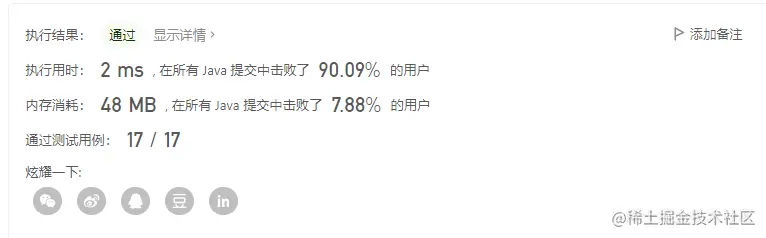# 剑指Offer每日一题16、调整奇偶顺序

### 二、题目

 输入：nums = [1,2,3,4]
输出：[1,3,2,4]
注：[3,1,2,4] 也是正确的答案之一。

1. 0 <= nums.length <= 50000
2. 1 <= nums[i] <= 10000

### 三、解题

#### 3.1 代码

 static public int[] exchange(int[] nums) {
int [] a=new int[nums.length];
int length = nums.length;
int j=0,k=0;
for (int i = 0; i < length; i++) {
if(nums[i]%2!=0){
a[j++]=nums[i];
}else{
k++;
a[length-k]=nums[i];
}
}
return a;
}

#### 3.2 执行效果• 时间复杂度 O（n）
• 空间复杂度 O（n）